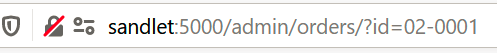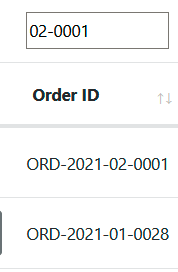# Initial filter set

Hi all,
I'm working on a initialization of the table filter. Filter values are to be passed in URL. The function looks like that:

``````function init_table_filter(table) {
var params = new URLSearchParams(window.location.search);
params.forEach((value, key) => {
var column = table.api().column(key + ':name');
\$('td:nth-child(' + (column.index() + 1) + ') input',
.val(value).trigger('change');
\$('td:nth-child(' + (column.index() + 1) + ') select',
.val(value).trigger('change');
});
}
``````

Filter applying function is defined like that:

``````function init_search_input(target, column) {
\$(target).on('keyup change clear', function () {
if ( column.search() !== this.value ) {
column
.search( this.value )
.draw();
}
});
}
``````

I made sure the columns where initial filter can be set have `name` property. When the page is loaded I see the filter value is set to the search field and `change` event is triggered and event handler is called. However no filtering occurs.If I type filter value after the page is loaded filtering works just fine.
How can I enforce it at page load?

This blog post here should help, it's discussing this issue.

Colin

• So if I understand it correctly the idea is to initialize search in table creation object, not later? I can deal with initialization of search fields separately. And as for column wise filtering that can be initialized in same way?

``````searchCols: [
null,
null,
null,
null,
null,
null,
null,
null,
{search: 'po_created'},
null
]
``````
• Ok, I figured out what was wrong with my code.
I had search initialized like this:

``````        initComplete: function() {
init_search(this, g_filter_sources)
init_table_filter(this);
}
``````

The `init_search()` function initializes column wise search with inputs for each column:

``````function init_search(table, filter_sources) {
table.api().columns().every(function() {
column = this;
\$('td:nth-child(' + (this.index() + 1) + ') input',
.each((_idx, item) => init_search_input(item, column))
.val('');
\$('td:nth-child(' + (this.index() + 1) + ') select',
.each((_idx, item) => init_search_select(
item, column, filter_sources[column.dataSrc()]))
.val('');
});
}
``````

The problem was that `init_search()` function executed asynchronously and `init_table_filter()` didn't wait for one. So attempt to set initial filter was made before the searching capability was actually established.

So I've remade search capability initialization this way:

``````function init_search(table, filter_sources) {
var promise = \$.Deferred();
var columns_left = table.api().columns().count();
table.api().columns().every(function() {
column = this;
\$('td:nth-child(' + (this.index() + 1) + ') input',
.each((_idx, item) => init_search_input(item, column))
.val('');
\$('td:nth-child(' + (this.index() + 1) + ') select',
.each((_idx, item) => init_search_select(
item, column, filter_sources[column.dataSrc()]))
.val('');
columns_left--;
if (!columns_left) {
promise.resolve();
}
});
return promise;
}
``````

and then set initial filter this way:

``````        initComplete: function() {
var table = this;
init_search(table, g_filter_sources)
.then(() => init_table_filter(table));
}
``````